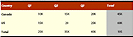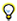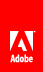# To perform calculations in a table

 You can use the sum function in FormCalc to total the values in a column. You must add the calculation to the footer row of the table. View full size graphicSelect the cell in the footer row where you want the calculation. For example, select the cell that corresponds to the total for Q1. In the Script Editor, select Calculate from the Show list. In the Language list, select FormCalc. In the Run At list, select Client. In the Script Source field, insert your FormCalc calculation. For example, to calculate the total for the Q1 data, type the following expression: `sum (Table.Row[*].Q1[*])` Repeat for the totals for Q2 and Q3. To calculate the totals for the country, type the following expression: `sum(Q1 + Q2 + Q3)` Repeat for the remaining row totals. For the grand total, type the following expression: `sum(TotalQ1 + TotalQ2 + TotalQ3)`To show the data as 10K, in the Object palette, click the Cell tab, click Patterns, and type z9'K' in the Pattern box. In the Value tab, select Calculated - Read Only from the Type list.Twitter™ and Facebook posts are not covered under the terms of Creative Commons.// Ethnio survey code removed